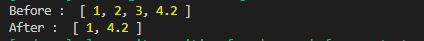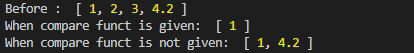Open In App

# Lodash _.differenceBy() Method

The _.differenceBy() method is used to remove the values from the original array by iterating over each element in the array by using the Iterate function. It is almost same as _.difference() function.

Syntax:

`lodash.differenceBy(array, [values], [iterate=_.identity])`

Parameters: This function accepts three parameters as mention above and describe below

• array: This is the array from which the values are to be removed.
• values: It is the Array of values that is to be removed from the original array.
• Iterate: This is the function that iterate over each element.

Note: If the iterate function is not given then _.differenceBy() function act as _.difference() function.

Return Value: This function returns an array.

Example 1:

## Javascript

 `// Requiring the lodash library``const _ = require(``"lodash"``);` `// Original array``let array1 = [1, 2, 3, 4.2]` `// Array to be subtracted``let val = [2, 3, 3, 5]` `// New Array after _.differenceBy()``// method where Math.double is the``// comparable function``let newArray = _.differenceBy(``    ``array1, val, Math.double);` `// Printing the original array``console.log(``"Before : "``, array1);` `// Printing the output``console.log(``"After : "``, newArray);`

Output:Example 2:

## Javascript

 `// Requiring the lodash library``const _ = require(``"lodash"``);` `// Original array``let array1 = [1, 2, 3, 4.2]` `// Value array to be subtracted``let val = [2, 3, 4, 5]` `// new Array after _.differenceBy()``// method where Math.double is the``// comparable function``let newArray1 = _.differenceBy(``    ``array1, val, Math.floor);` `// New Array after _.differenceBy function``// where no comparable function is given``let newArray2 = _.differenceBy(array1, val);` `// Printing the original array``console.log(``"Before : "``, array1);` `// printing the output``console.log(``"When compare funct is given: "``,``        ``newArray1);` `// Printing the output``console.log(``"When compare funct is not given: "``,``        ``newArray2);`

Output: